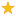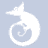quizzes  Forums# SAS DO loops

created by Tomowsiak on 23 Mar 12, enabled by AlexHard level (43% of success)    10 questions - 492 players
Quiz on do loops from the book1
Which statement is false regarding "do" loops?

2
How many times does this code run? data work. earnings; amount=1000; rate=0. 075/12; do month=1 to 12; amount+amount*rate; format amount dollar12. ; output; end; run;

3
If 5000 is invested on Jan 1 of each year for 15 years, complete the data step so as to determine the value of the account after 15 years at a constant 10 percent return rate.

4
What would be the stored value for year in the following? do year=1999 to 2004; capital+5000;capital+capital*. 1;end;run;

5
Which of the following is false regarding this code? data work. invest; do year=1990 to 2004; capital+5000;capital+capital*. 1;output;end;run;

6
How many observations will there be in work. earn? data work. earn; value=2000; do year=1 to 20; interest=value*0. 075; value+interest;output;end;run;

7
Which line generates the same records as this code? do until(capital gt 500000);

8
Complete the statement so that the program stops generating observations when distance is 250 miles or when 10 gallons of fuel have been used?

9
Which would be used to compare the result of investing 4000 a year for five years in three differnt banks that compound interest monthy?

10
Do loops can be used to sample data in a random way ?